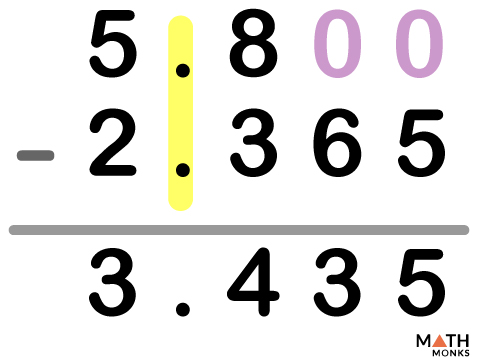# Subtracting Decimals

Certain parameters we come across in our daily lives such as medicines, chemicals, or currencies,are not whole numbers. They are decimals. However, we still do the calculations with such numbers. We will learn how to subtract decimal numbers here. An example is shown below where we will subtract like decimals.

## Steps for Subtracting Decimals

An exmaple is shown in the diagram below where we involve three simple steps while subtracting two like decimals (no. of digits after the decimal point is same).

Let us learn the steps for subtracting a decimal number from another decimal number.

1. Write down the decimal numbers one under the other in columns, such that their decimals points are lined up.
2. Put extra zeroes at the empty places to make the length of the numbers the same if needed.
3. Subtract in columns as usual.
4. Write down the result below. Drag the decimal point to the same position in the difference.

## Subtracting Decimals with Regrouping

Let us subtract two decimal numbers 5.64 and 3.89. Here, 9 is greater than 4 at the hundredth place. So we will borrow 1 from 6 at the tenth place. So we will mentally subtract 9 from 14 instead of 4. The diagram shows the method.

We will try another subtraction. Here we will do the regrouping mentally without writing the carry overs.

Let us learn to subtract two unlike decimals.

## Subtracting Unlike Decimals

Here we will subtract two unlike decimals with different lengths involving all the four steps mentioned above in this chapter. The numbers have different number of decimal places after the decimal point.

In the above diagram, we also involved the regrouping techniques mentally wherever needed. Whenever, there are zeros in the subtrahends, we regroup as per requirement.

Another example is shown below where the greater decimal number has the shorter length. The smaller decimal has more digits after the decimal point.

There is no need to put zeroes if the decimals are of the same length, i.e., the number of digits after the decimal points are the same.

## Subtracting Whole Numbers and Decimals

### A Decimal from a Whole Number

Another example is shown where we subtract a decimal from a whole number.

### A Whole Number from a Decimal

Another example is shown where we subtract a whole number from a decimal.

Let us learn how to subtract decimals with regrouping with a simple example.

## Solved Examples

25.75 – 18.42

Solution:

First, we will write the numbers in columns with decimal points.
25.75 – 18.42
The length of the two numbers is the same. So, no zero is needed.
We will simply subtract.
25.75 – 18.42
= 7.33

5.8 – 2.365Solution:

First, we will write the numbers in columns with decimal points.
The length of the numbers is not same. We will put zeros where needed.
5.800 – 2.365
= 3.435

15 – 12.42

Solution:

First, we will write the numbers in columns with aligning points.
We will put zeroes wherever needed in the whole number 15.
15. 00 – 12.42
= 2.58

32.109 – 26

Solution:

Writing the numbers in columns with the points lined up.
We will put zeroes wherever needed.
32.109 – 26.000
= 6.109